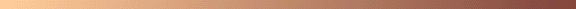ANALYSIS OF THE CHANCES IN FINDING
PALINDROMIC SQUARES AND CUBES```I had an attempt at finding a palindromic cube a couple of years ago
but with no success. I recently dusted it off and tried again still
with no success. I then thought I'd try to analyse what my chances
were. The following summarises what I found. I think the basic maths
is OK, but there may be some arithmetic errors.

I first looked at odd palindromic squares.
I am ignoring solutions of a special form eg those with just ones
and zeroes and assuming the problem is effectively random.

Starting with a specific example I looked at 17 digit numbers. The
range of such numbers is from 10^16 to 10^17 - 1. I ignored the
minus 1 for simplicity. Square roots ending in zero are not applicable
in this case so only 0.9 of roots are valid. Thus the number of square
roots in the range is approximately
0.9 * (sqrt(10^17) - sqrt(10^16)) = 1.95 * 10^8

The number of palindromic numbers in the target range is 10^9, but
only those ending in 1, 4, 5, 6 and 9 are of interest so there are
0.5 * 10^9 candidates. So the probability that a given perfect square
is also palindromic is 0.5 * 10^9/(10^17 - 10^16) which is
approximately 5.56 * 10^-9.
Thus the average density of palindromic squares is
5.56 * 10^-9 * 1.95 * 10^8 = 1.084

It seems to assume the distribution of palindromic squares is a Poissin
distribution which gives the probability of zero = exp(-1.0725) = .342
so the probability of 1 or more in the range is approximately .658.

It is quite easy to generalise this to for any odd digit range 2n + 1.
The range of numbers is 10^2n to 10^(2n + 1) - 1

The number of perfect squares is
0.9 * (sqrt(10^(2n + 1)) - sqrt(10^2n))
= 0.9 * sqrt(10^2n) * (sqrt(10) - 1) = 1.95 * 10^n

The number of palindromes is 0.5 * 10^(n + 1).

Thus the density of palindromic squares is
0.5 * 10^(n + 1) * 1.95 * 10^n / (10^(2n + 1) - 10^2n)
= 0.5 * 10 * 1.95 * 10^2n / 9 * 10^2n
= 1.084 and the probability of 1 or more palindromic squares in the
range is .658 .

This produces the interesting result that the probability of finding a
palindromic square of any specific length is constant. Of course they
get more difficult to find as the number of values that have to be
tested increases as the length of the number increases.

A similar result can be obtained for even length palindromic squares.
In this case I'll go straight to the general case.

For numbers with 2n digits the range is 10^(2n - 1) to 10^(2n) - 1

The number of perfect squares is
0.9 * (sqrt(10^(2n)) - sqrt(10^2n - 1))
= 0.9 * sqrt(10^2n) * (1 - sqrt(0.1)) = .615 * 10^n

The number of palindromes is 0.5 * 10^(n).

Thus the density of palindromic squares is
0.5 * 10^(n) * .615 * 10^n / (10^(2n) - 10^(2n - 1))
= 0.5 * 0.615 / .9
= .710 and the probability of 1 or more palindromic squares in the
range is .508 .

So an even-digit palindromic square is less probable than an odd-digit
palindromic square. This is not surprising because with odd digit
palindromes the middle digit is effectively a "free" digit which
can take any value from 0 to 9.

Now on to palindromic cubes. Once again I'll go straight to the
general case starting with odd-digit palindromes. In this case
palindromes can end in any digit except 0.

The range of numbers is 10^2n to 10^(2n + 1) - 1

The number of perfect cubes is
0.9 * (cuberoot(10^(2n + 1)) - cuberoot(10^2n))
= 0.9 * 10^(2n/3) * (cuberoot(10) - 1) = 1.039 * 10^(2n/3)

The number of palindromes is 0.9 * 10^(n + 1).

Thus the density of palindromic cubes is
1.039 * 10^(2n/3) * 0.9 * 10^(n + 1) / (10^(2n + 1) - 10^2n)
= (1.039 * 0.9 / 9) * 10^(2n/3) * 10^(n + 1) * 10^(-2n)
= .1039 * 10^(1 - n/3)

Notice this depends on 'n', so the density of possible solutions
decreases as numbers get larger. Thus for n = 8 ie length 17
we have density = .00223 and the probabilty of 1 or more
palindromic cubes in the range is 0.022 .
For n = 20 ie length 41 we have density = 2.24 * 10^-7 and the
probabilty of 1 or more palindromic cubes in the range is
2.24 * 10^-7 .

The conclusion is that the probability of finding a palindromic
cube is remote and the situation gets worse for higher powers.Mike Bennett (email) [ May 21, 2000 ].

```